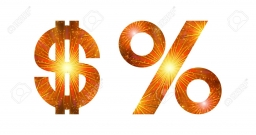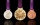# Unknown number

15% of the unknown number is 18 less than 21% of the same number. What is the unknown number?

Correct result:

x =  300

#### Solution:

0.15x + 18 = 0.21 x

0.15•x + 18 = 0.21•x

0.06x = 18

x = 300

Calculated by our simple equation calculator.Our examples were largely sent or created by pupils and students themselves. Therefore, we would be pleased if you could send us any errors you found, spelling mistakes, or rephasing the example. Thank you!

Please write to us with your comment on the math problem or ask something. Thank you for helping each other - students, teachers, parents, and problem authors.Tips to related online calculators
Do you have a linear equation or system of equations and looking for its solution? Or do you have quadratic equation?

## Next similar math problems:

• Reducing numberReducing the an unknown number by 28.5% we get number 243.1. Determine unknown number.
• PercentCalculate how many % is the number 26.25 less than the number 105.
• Perctentages35% of what number is 35?
• CompetitorsIn the first round of slalom fell 15% of all competitors and in the second round another 10 racers. Together, 40% of all competitors fell. What was the total number of competitors?
• PupilsThere are 350 girls in the school, and the other 30% of the total number of pupils are boys. How many pupils does the school have?
• Jane classWhen asked how many students are in class, Jane said, if we increase the number of students in our class by hundred % and then add half the number of students, we get 100. How many students are in Jane's class?
• SummerjobThe temporary workers planted new trees. Of the total number of 500 seedlings, they managed to plant 426. How many percents did they meet the daily planting limit?
• Profit gainIf 5% more is gained by selling an article for Rs. 350 than by selling it for Rs. 340, the cost of the article is:
• Sale offThe TV went down 10% and then 10% off the original price again. Now it costs 300 €. What was its original price?
• Percentages52 is what percent of 93?
• Cinema ticketsCinema sold 180 tickets this Thursday, which is 20%. Monday 14%, Tuesday 6%, Wednesday 9%, Friday 24%, Saturday 12%, and Sunday 15%. How many tickets were sold per week?
• Art schoolEvery fifth pupil 9A goes to art school. How many percent of pupils in class 9A go to art school?
• PersonsPersons surveyed:100 with result: Volleyball=15% Baseball=9% Sepak Takraw=8% Pingpong=8% Basketball=60% Find the average how many like Basketball and Volleyball. Please show your solution.
• Highway repairThe highway repair was planned for 15 days. However, it was reduced by 30%. How many days did the repair of the highway last?
• The Chemistry testThe Chemistry test contained 8 questions, each with 3 points. Peter scored 21 points. How many percent did Peter write a test?
• WalkingOf the 450 students at the school, 432 walks to school. What percentage is this?
• RewardJanko and Peter divided the reward from the brigade so that Peter got 5/8 of the reward. What percentage of Janko's reward got?